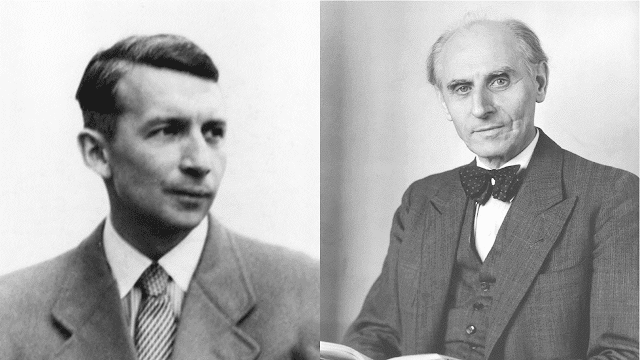### Notes on Ising modelErnst Ising (Left) and Wilhelm Lenz (Right) - Ising was Lenz's student and the Ising model was claimed (by Ising himself) to be originated by Lenz but named after Ising.

Ising model was originally proposed for ferromagnetism and owing to its simplicity in describing the system interaction, it has been widely used in not only describing magnetic systems in theoretical physics area but also in other areas involving similar interaction mechanism. In fact, Ising model can potentially find its position in describing systems involving binary states whereby we have active influence upon each other between neighbors. Here in this blog, I will not try to reproduce those already available beautiful introduction to Ising model - see e.g., Ref. [1-4] and a whole bunch of others one can easily find on Internet. Instead, I will just write down the outline of the problem we are facing once we have the simple Ising model set up for describing the system energy. For example, we always hear people talking about the exact solution for Ising model. But, what actually are we trying to solve? What about those non-exact ways for 'solving' the Ising model?

First, it is worthwhile to write down the Hamiltonian for Ising model,

$E_I{S_i} = -\sum_{\langle i,j\rangle}J_{ij}S_iS_j - \sum_{i=1}^NB_iSi$

where the subscript $$I$$ represents the Ising model. The first term corresponds to the interaction energy between neighboring spins and the second term is the interaction energy with externally applied magnetic field. $$\{S_i\}$$ is a shortcut for the spin configuration of the system where each spin on its lattice site has its own orientation - either up (+1) or down (-1). $$i$$ and $$j$$ refers to lattice site and $$J_{ij}$$ is the exchange constant describing the interaction strength between neighboring spins. Given the Hamiltonian of system, we are trying to calculate a crucial quantity - the partition function, which is a defined as the function of system state variables such as temperature, volume, etc. Starting from partition function, we can derive most of other thermodynamic variables, e.g., system total energy, free energy, entropy, pressure, etc. Then naturally we can look into the phase transition problem, e.g., for magnetic system, using those quantities derived from partition function. Therefore, for Ising model, when we say we want the exact solution, we mean to obtain the exact analytical solution for the partition function, the general expression of which is given as below,

$Z = \sum_{s_1=-1}^{+1}\sum_{s_2=-1}^{+1}\cdots\sum_{s_N=-1}^{+1}e^{-\beta E_I\{S_i\}}$

The solution for 1D Ising model can be found in Ref. , which is based on the original publication by Kramers and Wannier . The exact solution for 2D model was originally proposed by Onsager [6-8] and the whole derivation procedure is reproduced in details as presented in Ref. . The exact solution for 3D situation has been a challenging question to the physics community. In 2007, Prof. Z. Zhang proposed his exact solution based on two conjectures  and currently it is still under active debate and development [10, 11]. About this debate, Prof. Z. Zhang has three series of blogs introducing the history and current development of this theory - one can refer to Ref. [12-14] for details.

Apart from exact solution, there are other ways to explore the phase transition behavior using Ising model, mainly based on numerical or simulation (e.g., Monte Carlo) approach. An approach based on iterative numerical technique can be found in Ref.  and typical examples for those based on Monte Carlo simulation can be found in Ref. [15, 16].

References